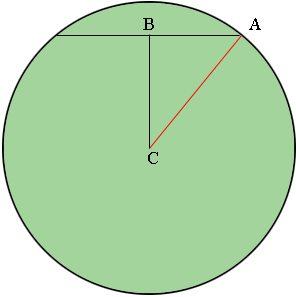SEARCH HOMEMath Central Quandaries & QueriesQuestion from Sophia, a student: The diagram shows a circle with a chord that is 10cm long. The middle of the chord is 12cm from the centre of the circle. Calculate the radius. Chord length is 10cm. The distance from the centre to chord is 12 cm.Hi Sophia,

The subject line of your message to us was "Pythagoras theorem". That says it all!I added one line to the diagram you described and labeled some points. B is the midpoint of the chord and C is the centre of the circle. Angle ABC is a right angle. Do you see why? Thus triangle ABC is a right triangle. What does Pythagoras theorem tell you?

PennyMath Central is supported by the University of Regina and The Pacific Institute for the Mathematical Sciences.